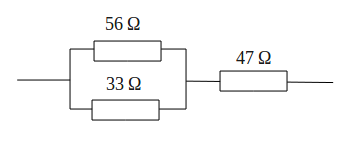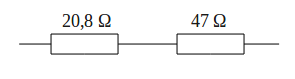# How to calculate mixture circuit (series and parallel) on the resistors?

In a the circuit mixes, some of the resistors are connected in series while the other is connected in parallel. To calculate the value of Rt in series-parallel circuit, the first method is to find the groups of resistors are all connected in series or all of which are connected in parallel.

Draw back the circuit, by replacing each group of resistors into an equivalent resistor. Gradually, simplify the circuit to produce an Rt, or the resistance of a single end.

Example question:
What is the value of Rt, or the equivalent resistance of the circuit resistor mixture below?Completion:
On the image above the resistance value of 56 Ω and 33 Ω are connected in parallel, then parallel connection is connected in series with 47 Ω.

Create Rt1 equivalent resistance for parallel connection resistance 56 Ω and 33 Ω, with a parallel formula
1/Rt = 1/R1 + 1/R2 + 1/R3 + · · · + 1/Rn, then
1/Rt1 = 1 / 56 + 1 / 33
1/Rt1 = 89/1848
89Rt1 = 1848
Rt1 = 1848/89
Rt1 = 20.8 Ω

Parallel connection of resistance 56 Ω and 33 Ω can be replaced with an equivalent resistance of 20.8 Ω. This simplification produces two resistance are connected in series, which is 20.8 Ω and 47 Ω.Equivalent series resistance value according to the series formula
Rt = R1 + R2 + R3 + · · · + Rn, then
Rt = 20.8 + 47
Rt = 68.7 Ω

Thus obtained Rt or an equivalent resistance for 67.8 ΩNext→
←Prev